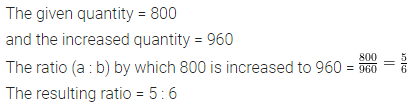# Selina Concise Mathematics Class 6 ICSE Solutions Chapter 11 Ratio

## Selina Concise Mathematics Class 6 ICSE Solutions Chapter 11 Ratio

Selina Publishers Concise Mathematics Class 6 ICSE Solutions Chapter 11 Ratio

### Ratio Exercise 11A – Selina Concise Mathematics Class 6 ICSE Solutions

Question 1.
Express each of the following ratios in its simplest form :
(a) (i) 4 : 6
(ii) 48 : 54
(iii) 200 : 250
(b) (i) 5 kg : 800 gm
(ii) 30 cm : 2 m
(iii) 3 m : 90 cm
(iv) 2 years : 9 months
(v) 1 hour : 45 minutes
(vi) 4 min : 45 sec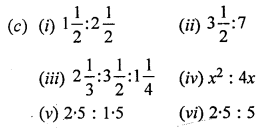Solution: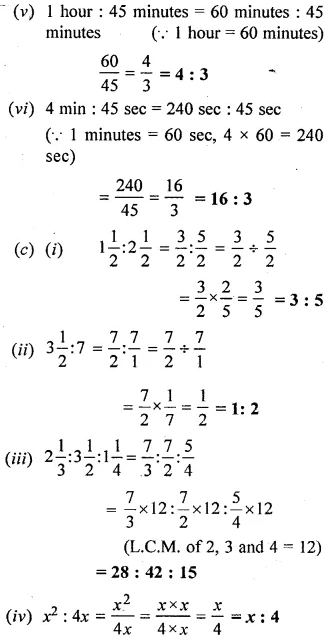Question 2.
A field is 80 m long and 60 m wide. Find the ratio of its width to its length.
Solution:Question 3.
State, true or false :
(i) A ratio equivalent to 7 : 9 is 27 : 21.
(ii) A ratio equivalent to 5 : 4 is 240 : 192.
(iii) A ratio of 250 gm and 3 kg is 1 : 12.
Solution:Question 4.
Is the ratio of 15 kg and 35 kg same as the ratio of 6 years and 14 years?
Solution:Question 5.
Is the ratio of 6 g and 15 g same as the ratio of 36 cm and 90 cm?
Solution: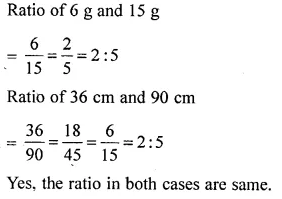Question 6.
Find the ratio between 3.5 m, 475 cm and 2.8 m.
Solution:Question 7.
Find the ratio between 5 dozen and 2 scores. [1 score = 20]
Solution:### Ratio Exercise 11B – Selina Concise Mathematics Class 6 ICSE Solutions

Question 1.
The monthly salary of a person is Rs. 12,000 and his monthly expenditure is Rs 8,500. Find the ratio of his:
(i) salary to expenditure
(ii) expenditure to savings
(iii) savings to salary
Solution: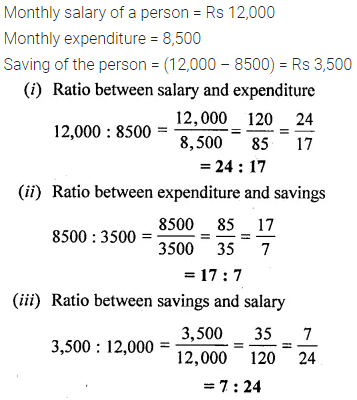Question 2.
The strength of a class is 65, including 30 girls. Find the ratio of the number of:
(i) girls to boys
(ii) boys to the whole class
(iii) the whole class to girls.
Solution: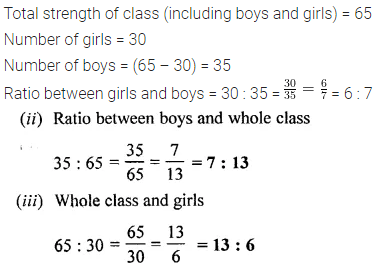Question 3.
The weekly expenses of a boy have increased from ₹ 1,500 to ₹ 2,250. Find the ratio of:
(i) increase in expenses to original expenses.
(ii) original expenses to increased expenses.
(iii) increased expenses to increase in expenses.
Solution: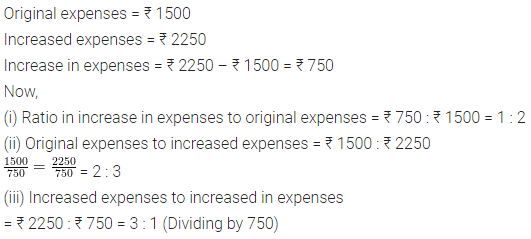Question 4.
Reduce each of the following ratios to their lowest terms :
(i) 1 hour 20 min : 2 hours
(ii) 4 weeks : 49 days
(iii) 3 years 4 months : 5 years 5 months.
(iv) 2 m 40 cm : 1 m 44 cm
(v) 5 kg 500 gm : 2 kg 750 gm
Solution: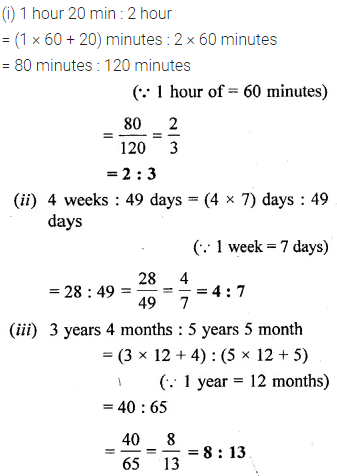Question 5.
Two numbers are in the ratio 9 : 2. If the smaller number is 320, find the larger number.
Solution:Question 6.
A bus travels 180 km in 3 hours and a train travels 450 km in 5 hours. Find the ratio of speed of train to speed of bus.
Solution:Question 7.
In winters, a school opens at 10 a.m. and closes at 3.30 p.m. If the lunch interval is of 30 minutes, find the ratio of lunch interval to total lime of the class periods.
Solution: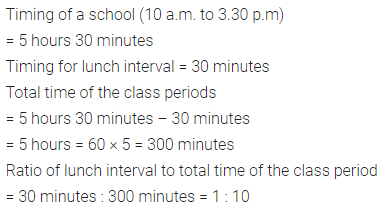Question 8.
Rohit goes to school by car at 60 km per hour and Manoj goes to school by scooty at 40 km per hour. If they both live in the same locality, find the ratio between the time taken by Rohit and Manoj to reach school.
Solution: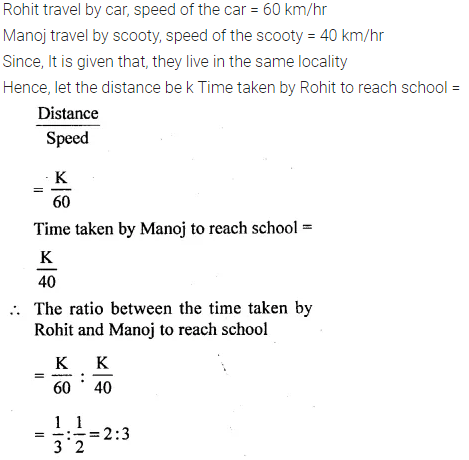Question 9.
In a club having 360 members, 40 play carrom, 96 play table tennis, 144 play badminton and remaining members play volley-ball. If no member plays two or more games, find the ratio of members who play :
(i) carrom to the number of those who play badminton.
(ii) badminton to the number of those who play table-tennis.
(iii) table-tennis to the number of those who play volley-ball.
(iv) volleyball to the n umber of those who play other games.
Solution: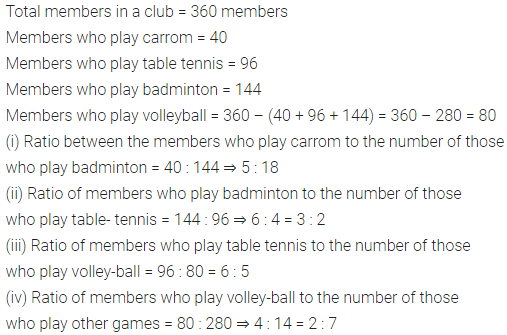Question 10.
The length of a pencil is 18 cm and its radius is 4 cm. Find the ratio of its length to its diameter.
Solution:Question 11.
Ratio of distance of the school from A’s home to the distance of the school from B’s home is 2 : 1.
(i) Who lives nearer to the school?
(ii) Complete the following table :Solution: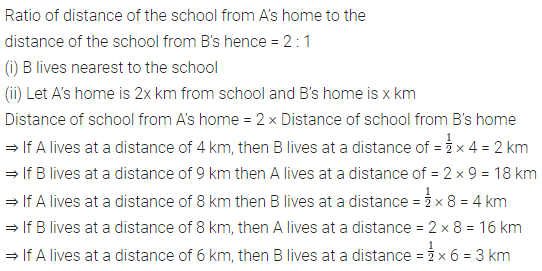Question 12.
The student-teacher ratio in a school is 45 : 2. If there are 4050 students in the school, how many teachers must be there?
Solution: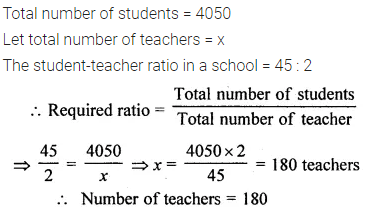### Ratio Exercise 11C – Selina Concise Mathematics Class 6 ICSE Solutions

Question 1.
₹ 120 is to be divided between Hari and Gopi in the ratio 5 : 3. How much does each get?
Solution:Question 2.
Divide 72 in the ratio $$2\frac { 1 }{ 2 } :1\frac { 1 }{ 2 }$$
Solution: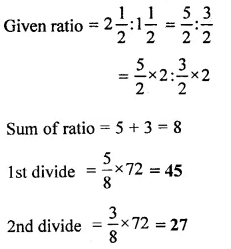Question 3.
Divide 81 into three parts in the ratio 2 : 3 : 4.
Solution: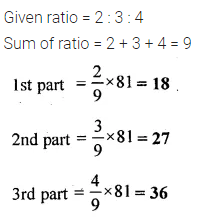Question 4.
Divide Rs 10,400 among A, B and C in the ratio $$\frac { 1 }{ 2 } :\frac { 1 }{ 3 } :\frac { 1 }{ 4 }$$
Solution: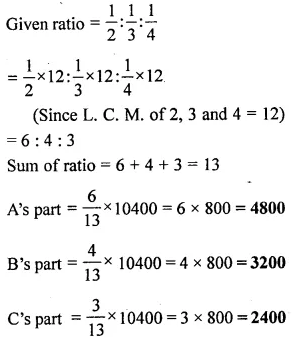Question 5.
A profit of Rs 2,500 is to be shared among three persons in the ratio 6 : 9 : 10. How much does each person get?
Solution:Question 6.
The angles of a triangle are in the ratio 3 : 7 : 8. Find the greatest and the smallest angles.
Solution: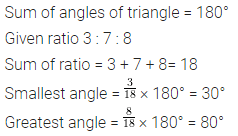Question 7.
The sides of a triangle are in the ratio 3 : 2 : 4. If the perimeter of the triangle is 27 cm, find the length of each side.
Solution:Question 8.
An alloy of zinc and copper weighs 12$$\frac { 1 }{ 2 }$$ kg. if in the alloy, the ratio of zinc and copper is 1 : 4, find the weight of copper in it.
Solution: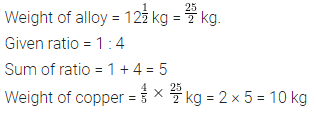Question 9.
How will Rs 31500 be shared between A, B and C ; if A gets the double of what B gets, and B gets the double of what C gets?
Solution: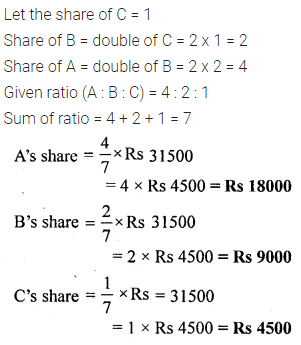Question 10.
Mr. Gupta divides Rs 81000 among his three children Ashok, Mohit and Geeta in such a way that Ashok gets four times what Mohit gets and Mohit gets 2.5 times what Geeta gets. Find the share of each of them.
Solution: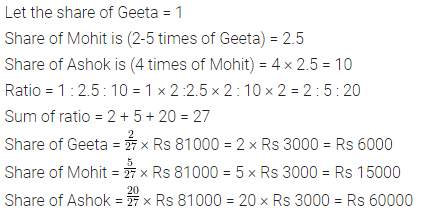### Ratio Exercise 11D – Selina Concise Mathematics Class 6 ICSE Solutions

Question 1.
Which ratio is greater:

Solution:Question 2.
Which ratio is smaller :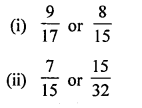Solution: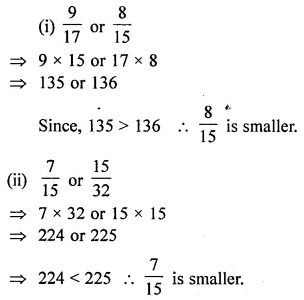Question 3.
Increase 95 in the ratio 5 : 8.
Solution: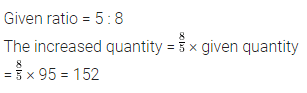Question 4.
Decrease 275 in the ratio 11 : 7.
Solution: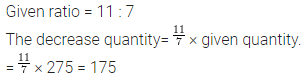Question 5.
Decrease 850 in the ratio 17 : 6 and then increase the result in the ratio 5 : 9.
Solution:Question 6.
Decrease 850 in the ratio 17 : 6 and then decrease the resulting number again in 4 : 3.
Solution:Question 7.
Increase 1200 in the ratio 2 : 3 and then decrease the resulting number in the ratio 10 : 3.
Solution:Question 8.
Increase 1200 in the ratio 3 : 7 and then increase the resulting number again in the ratio 4 : 7.
Solution: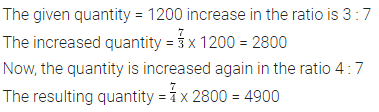Question 9.
The number 650 is decreased to 500 in the ratio a : b, find the ratio a : b.
Solution: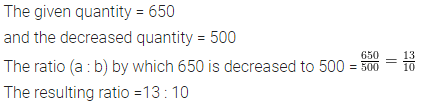Question 10.
The number 800 is increased to 960 in the ratio a : b, find the ratio a : b.
Solution: